# Data Table Data Table Object A Data Table

• Slides: 16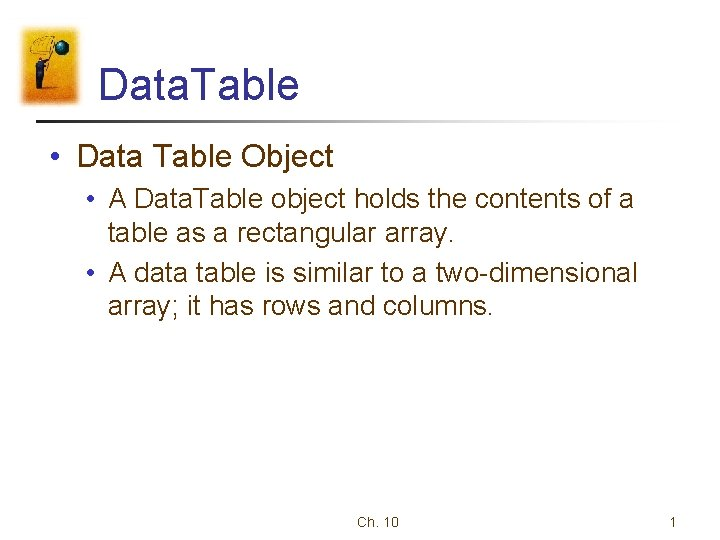Data. Table • Data Table Object • A Data. Table object holds the contents of a table as a rectangular array. • A data table is similar to a two-dimensional array; it has rows and columns. Ch. 10 1Important Note • Two steps for each program in order to gain access to the Data Table object. 1. Add references to System. Data. dll and System. Xml. dll. (Next slide) 2. Type the statement Imports System. Data at the top of the code window. Ch. 10 2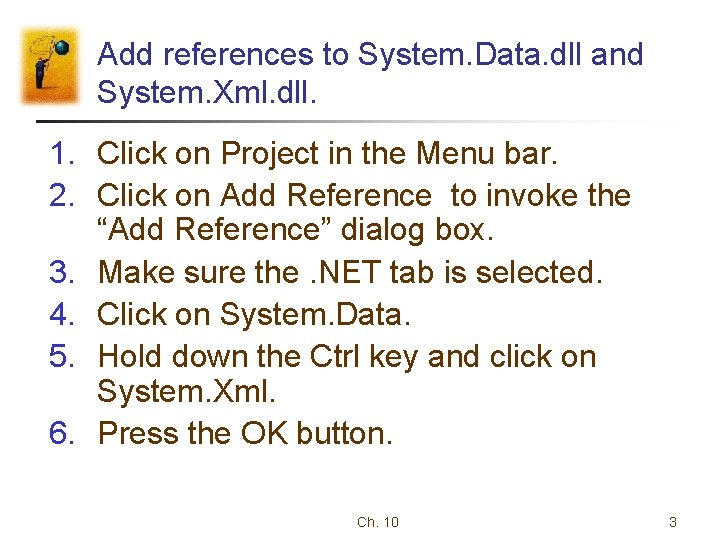Add references to System. Data. dll and System. Xml. dll. 1. Click on Project in the Menu bar. 2. Click on Add Reference to invoke the “Add Reference” dialog box. 3. Make sure the. NET tab is selected. 4. Click on System. Data. 5. Hold down the Ctrl key and click on System. Xml. 6. Press the OK button. Ch. 10 3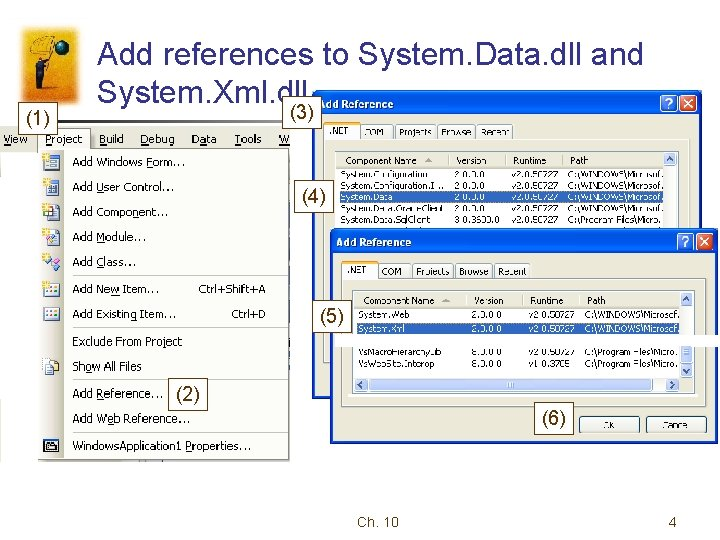(1) Add references to System. Data. dll and System. Xml. dll. (3) (4) (5) (2) (6) Ch. 10 4Data Adapter • A data adapter is an intermediary object that serves as a channel to allow bidirectional data transfers between database and data. Table or data. Set Database Data Adapter Data. Table Ch. 10 5Data. Table Variable • • We will assume that the two steps discussed in the previous slide (1. add references; 2. Imports system. data) have been carried out for every program in this chapter. Then, the following declares a Data. Table variable Dim dt As New Data. Table() Ch. 10 6Fill method • The Fill method of the Data. Adapter fills a Data. Set or datatable with data retrieved from a query • For example, Dim dt as New data. Table() … data. Adapter. fill(dt) Ch. 10 7Dispose Method • It releases all resources used by the Data. Adapter. • For example, Dim dt as New Data. Table() … data. Adapter. Fill(dt) ‘filling data into dt ‘release all resource used by data. Adapter. Dispose() Ch. 10 8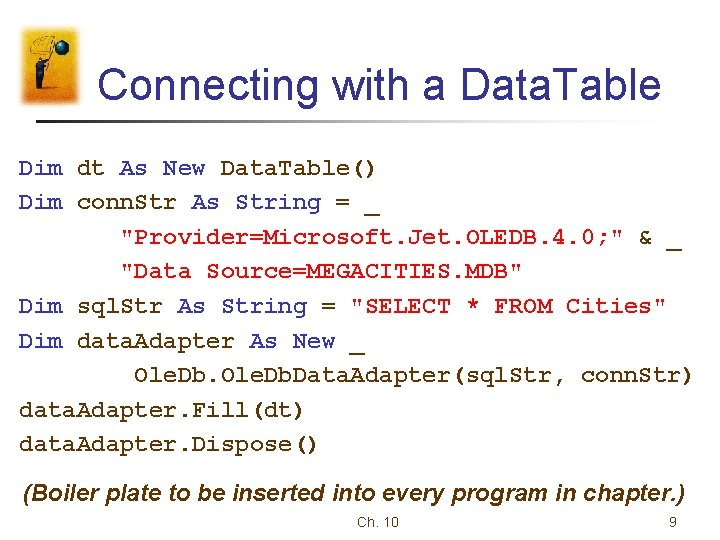Connecting with a Data. Table Dim dt As New Data. Table() Dim conn. Str As String = _ "Provider=Microsoft. Jet. OLEDB. 4. 0; " & _ "Data Source=MEGACITIES. MDB" Dim sql. Str As String = "SELECT * FROM Cities" Dim data. Adapter As New _ Ole. Db. Data. Adapter(sql. Str, conn. Str) data. Adapter. Fill(dt) data. Adapter. Dispose() (Boiler plate to be inserted into every program in chapter. ) Ch. 10 9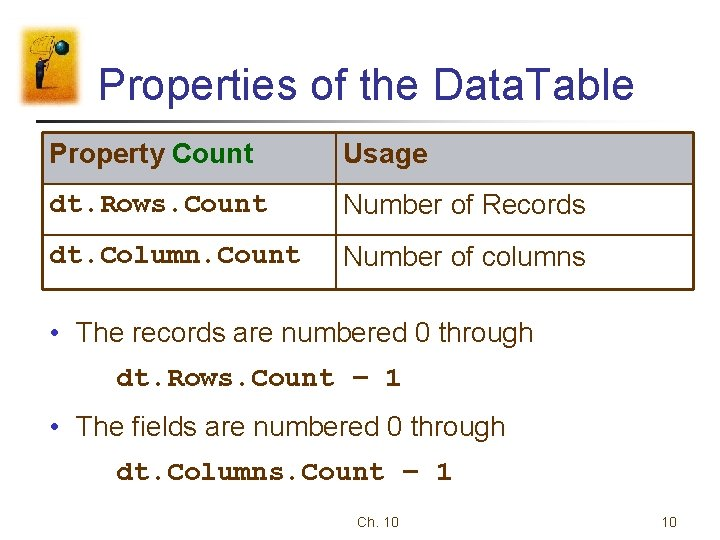Properties of the Data. Table Property Count Usage dt. Rows. Count Number of Records dt. Column. Count Number of columns • The records are numbered 0 through dt. Rows. Count – 1 • The fields are numbered 0 through dt. Columns. Count – 1 Ch. 10 10More Properties • The name of the jth field is given by dt. Columns(j) • The entry in the jth field of the ith record is dt. Rows(i)(j) • The entry in the specified field of the ith record is dt. Rows(i)(field. Name) Ch. 10 11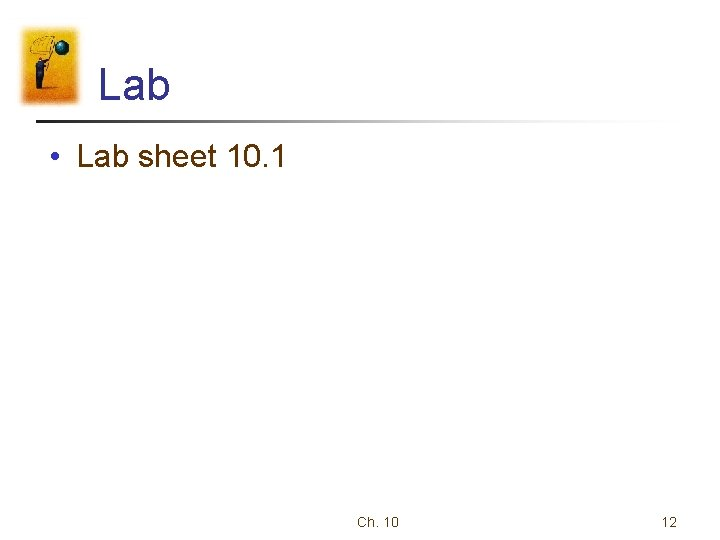Lab • Lab sheet 10. 1 Ch. 10 12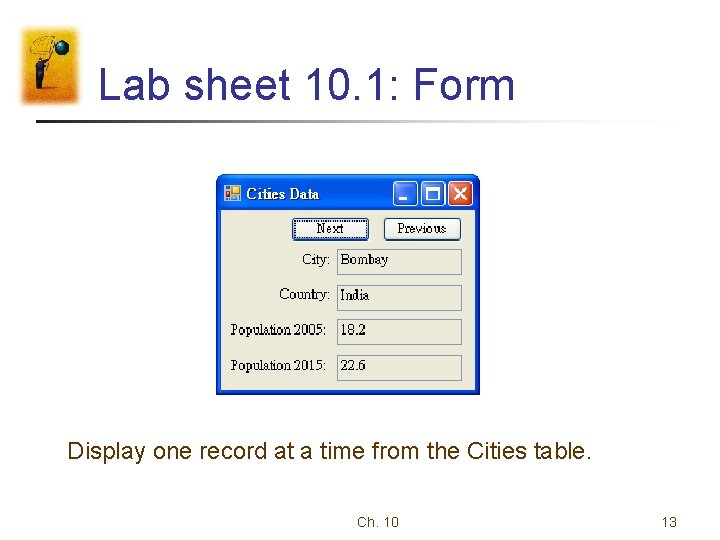Lab sheet 10. 1: Form Display one record at a time from the Cities table. Ch. 10 13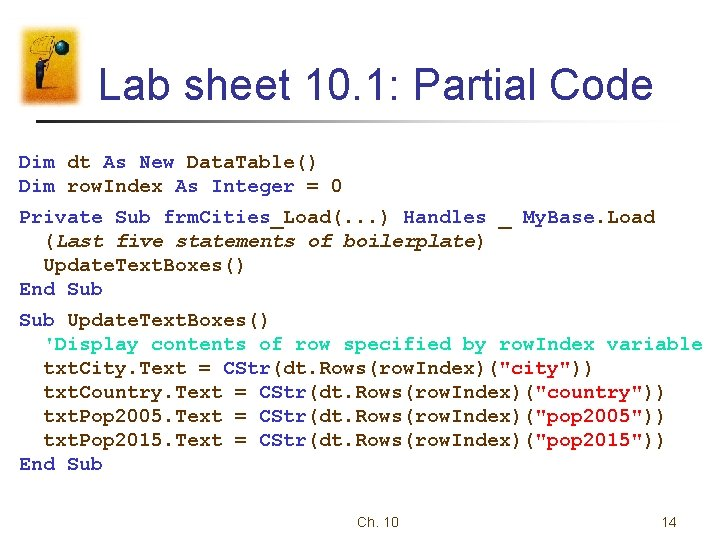Lab sheet 10. 1: Partial Code Dim dt As New Data. Table() Dim row. Index As Integer = 0 Private Sub frm. Cities_Load(. . . ) Handles _ My. Base. Load (Last five statements of boilerplate) Update. Text. Boxes() End Sub Update. Text. Boxes() 'Display contents of row specified by row. Index variable txt. City. Text = CStr(dt. Rows(row. Index)("city")) txt. Country. Text = CStr(dt. Rows(row. Index)("country")) txt. Pop 2005. Text = CStr(dt. Rows(row. Index)("pop 2005")) txt. Pop 2015. Text = CStr(dt. Rows(row. Index)("pop 2015")) End Sub Ch. 10 14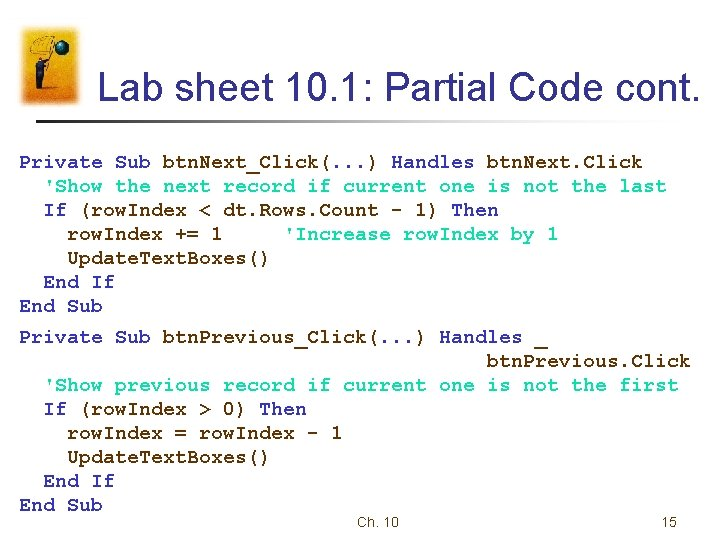Lab sheet 10. 1: Partial Code cont. Private Sub btn. Next_Click(. . . ) Handles btn. Next. Click 'Show the next record if current one is not the last If (row. Index < dt. Rows. Count - 1) Then row. Index += 1 'Increase row. Index by 1 Update. Text. Boxes() End If End Sub Private Sub btn. Previous_Click(. . . ) Handles _ btn. Previous. Click 'Show previous record if current one is not the first If (row. Index > 0) Then row. Index = row. Index - 1 Update. Text. Boxes() End If End Sub Ch. 10 15Lab sheet 10. 1: Output Ch. 10 16